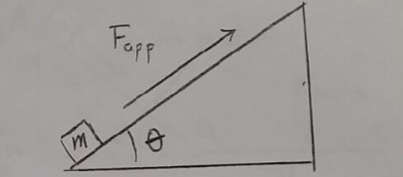# Problem: Consider the arrangement shown in the figure. A box of mass m = 5.00 kg is pushed up a slope of inclination angle θ = 25° by an applied force Fapp. The coefficients of static and kinetic friction between the box and the inclined plane are μs = 0.30 and μk = 0.20 respectively. Calculate the maximum static frictional force that can exist between the box and the inclined plane. Note that your result should be larger than your answer to part b. Using your result for the maximum static frictional force, determine the minimum Fapp required to get the box moving.

###### Problem Details

Consider the arrangement shown in the figure. A box of mass m = 5.00 kg is pushed up a slope of inclination angle θ = 25° by an applied force Fapp. The coefficients of static and kinetic friction between the box and the inclined plane are μs = 0.30 and μk = 0.20 respectively.

Calculate the maximum static frictional force that can exist between the box and the inclined plane. Note that your result should be larger than your answer to part b. Using your result for the maximum static frictional force, determine the minimum Fapp required to get the box moving.